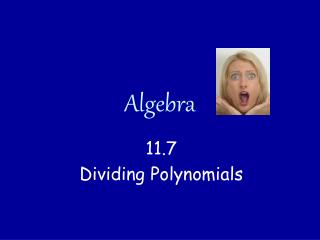DownloadDownload PresentationAlgebra

# Algebra

Télécharger la présentation## Algebra

- - - - - - - - - - - - - - - - - - - - - - - - - - - E N D - - - - - - - - - - - - - - - - - - - - - - - - - - -
##### Presentation Transcript

1. Algebra 11.7 Dividing Polynomials

2. Dividing a Polynomial by a Monomial Divide 9x² + 12x – 18 by 3x 3 4 9x² + 12x – 18 12x 6 9x² 18 = + - 3x 1 3x 1 3x 1 3x 6 = 3x + 4 - x 1. Divide out common factors. Numbers first. Variables next. 2. Rewrite in simplified form.

3. Long Division with Numbers The algorithm now seems simple. 2 3 28 658 Divide 658 by 28 Ask: how many 28’s in 65? About2 56 Multiply 2 x 28 and subtract from 65 98 Ask: how many 28’s in 98? About3 84 Multiply 3 x 28 and subtract from 98 14 Remainder is 14 14 Remainder Quotient: 23 + = 23½ 28 Divisor

4. Polynomial Long Division Polynomial long division uses the same process as with numbers. It is used to divide a polynomial by a binomial. -2 3x 3x² + 7x - 12 Divide 3x² + 7x – 12 by x + 3 x + 3 Ask: how many x’s in 3x²? 3x 3x² + 9x Multiply 3x by x + 3 and subtract Change signs when subtracting! -2x - 12 Ask: how many x’s in -2x? -2 -2x - 6 Multiply -2 by x + 3 and subtract -6 Remainder is -6 -6 Remainder Quotient: 3x - 2 + x + 3 Divisor

5. Let’s try one together. x - 5 x² - 3x + 5 Divide x²- 3x + 5 by x + 2 x + 2 Ask: how many x’s in x²? x x² + 2x Multiply x by x + 2 and subtract Change signs when subtracting! -5x + 5 Ask: how many x’s in -5x? -5 -5x - 10 Multiply -5 by x + 2 and subtract 15 Remainder is 15 Quotient:

6. You try. x + 3 x² + 2x + 4 Divide x²+ 2x + 4 by x - 1 x - 1 Ask: how many x’s in x²? x x² - x Multiply x by x - 1 and subtract Change signs when subtracting! 3x + 4 Ask: how many x’s in 3x? 3 3x - 3 Multiply 3 by x - 1 and subtract 7 Remainder is 7 Quotient:

7. Adding a Place-holding ZERO. Insert a place-holding 0x 6x - 12 6x² + 0x - 11 Divide 6x²- 11 by x + 2 x + 2 Ask: how many x’s in 6x²? 6x 6x² +12x Multiply 6x by x + 2 and subtract Change signs when subtracting! -12x - 11 Ask: how many x’s in -12x? -12 -12x -24 Multiply -12 by x + 2 and subtract 13 Remainder is 13 Quotient:

8. Homework pg. 687 #15-21 odd #27-45 odd